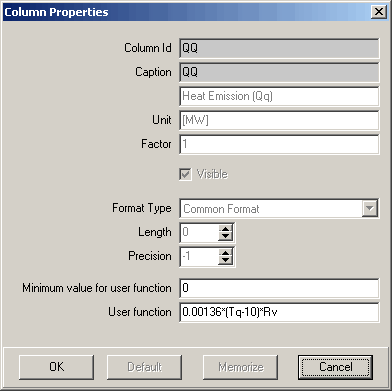# P&K ODOR

## Column PropertiesIn this form the properties of the column can be changed.

The column name shown here is an example only and possibly not available in your program.

### Column Id

This is the internal identifier of the column. If properties are stored via Memorize, those properties will be used for this identifier.

### Caption

Column title for the output.

### Unit

Here the unit can be changed, in order to adapt it e.g. to a factor.

### Factor

All values of the column are multiplied with this value before displayed.

### Format Type

The Format Type is available for real numbers only and has following meanings:

• Scientific Notation
• The value is converted into a string with the following form: " - d,ddd... E+ddd ". If it concerns a negative number, the string begins with a minus sign. There is always one digit before the decimal separator. The total number of the digits in the result string (including the digit before the decimal separator) is determined by the precision. If this is missing (-1), a given accuracy is assumed by 15 digits. The exponent character " E " is always followed by a plus or minus sign and at least three digits.
• Fixed Digit Number
• The value is converted into a string with the following form: " - ddd, ddd... ". If it concerns a negative number, the string begins with a minus sign. The number of places after the decimal comma is determined by the precision. If this is missing (-1), a given accuracy is used by two decimal places.
• Common Format
• The value is converted using the format fixed or scientific notation into the shortest possible decimal string. The number of significant places in the resulting string is determined by the precision. If this is missing (-1), a given accuracy is assumed by 15 places. Following zeros are removed from the resulting string. A decimal separator is displayed only if necessary. For the resulting string the fixed number format is used, if the number of places before the decimal separator is smaller or equal the precision and the value greater or equal to 0.00001. In all other cases the scientific notation is used.

See Format Type.

### Default

This button resets the settings to the factory defaults. The new settings will not become effective in all cases.

### Memorize

With this button, the current settings are memorized (for this program) and used in the next session.

Following might not be available in your program yet, or is solved in a different way (P&K 3950)

### Minimum value for User function

The User function will not be evaluated, if the cell value is not greater or equal to this value.

### User function

The User function can be defined to calculate values, depending on the values of other columns and the same row. Use the Column Id to address a column.

Language: